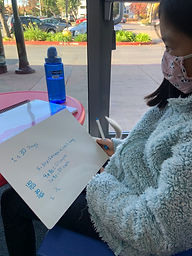Mr. Rodney

Target 1​

Lesson Type:

Review

Number Operation

:

Fractional Numbers

Convert between fractions, decimals, and percentages.

1:

Describe the relationship between fractions, decimals, and percentages.

2:

Convert fractions to decimals and decimals to fractions.

3:

Understand that the digit to the left of the decimal is the whole number, the digits to the right are the numerator, and the denominator is the place-value of the furthest right number.

5th

Vocabulary:

Fraction, Decimal, Place Value, Inequality, Lesser Than, Greater Than, Equal To

Activities:

• Students solved multi-step word problems dealing with ratio calculating price per pound, rounding to the nearest hundredth's place.
• Determined what fraction of a bag 2 dogs each ate in a month given the amount of cups fed per meal and the number of cups in each bag of dog food.
• Expressed answers in simplest form when necessary.
• Used inequality symbols to compare fractions and decimals.Home Exploration

Guiding Questions:Absent Students:

Ruhi

Target 2

:

1:

Use reflectional and rotational symmetry to identify and describe tessellations.

2:

Understand that a tessellation is a repeated geometric figure that covers a plane without gaps or overlaps.

3:

Construct a tessellation and use it to create a pattern in which there are no empty spaces.

Vocabulary:

Tessellations, Shapes, Congruent

Activities:

• Identified where tessellations exists in the real world.
• Copied tessellation figures and traced them to create a "tiling" effect.
• Explored how to create their own tessellations tiles.Home Exploration

Guiding Questions:Target 3

:

Vocabulary:

Activities:Home Exploration